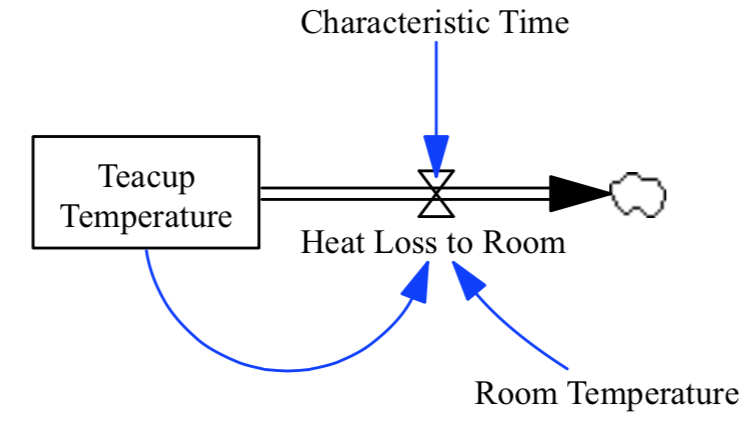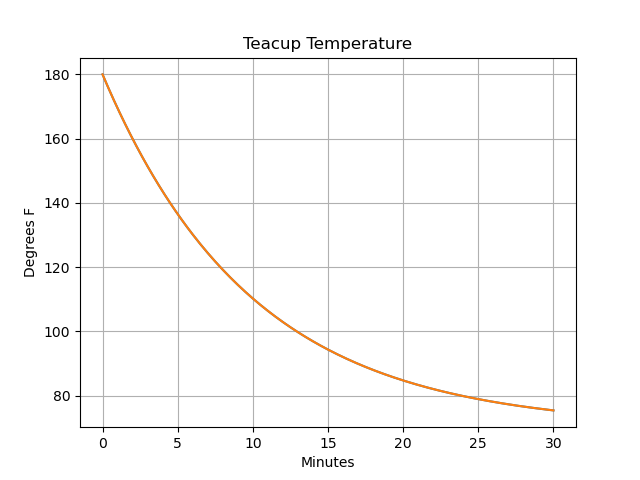Basic Usage¶

Importing a model and getting started¶

To begin, we must first load the PySD module, and use it to import a supported model file:

import pysd

This code creates an instance of the PySD class loaded with an example model that we will use as the system dynamics equivalent of ‘Hello World’: a cup of tea cooling to room temperature.To view a synopsis of the model equations and documentation, call the doc() method of the model class. This will generate a listing of all the model elements, their documentation, units, equations, and initial values, where appropriate. Here is a sample from the teacup model:

>>> print model.doc()

Note

You can also load an already translated model file, what will be faster as you will load a Python file:

import pysd

Note

The functions read_vensim(), read_xmile() and load() have optional arguments for advanced usage, you can check the full description in User Functions Reference or using help() e.g.:

import pysd

Running the Model¶

The simplest way to simulate the model is to use the run() command with no options. This runs the model with the default parameters supplied by the model file, and returns a Pandas dataframe of the values of the stocks at every timestamp:

>>> stocks = model.run()

t        teacup_temperature
0.000    180.000000
0.125    178.633556
0.250    177.284091
0.375    175.951387

Pandas gives us simple plotting capability, so we can see how the cup of tea behaves:

stocks.plot()
plt.ylabel('Degrees F')
plt.xlabel('Minutes')To show a progressbar during the model integration the progress flag can be passed to the run() command, progressbar package is needed:

>>> stocks = model.run(progress=True)

Running models with DATA type components¶

Venim’s regular DATA type components are given by an empty expression in the model equation. These values are read from a binary .vdf file. PySD allows running models with this kind of data definition using the data_files argument when calling run() command, e.g.:

>>> stocks = model.run(data_files="input_data.tab")

Several files can be passed by using a list, then if the data information has not been found in the first file, the next one will be used until finding the data values:

>>> stocks = model.run(data_files=["input_data.tab", "input_data2.tab", ..., "input_datan.tab"])

If a variable is given in different files to choose the specific file a dictionary can be used:

>>> stocks = model.run(data_files={"input_data.tab": ["data_var1", "data_var3"], "input_data2.tab": ["data_var2"]})

Note

Only tab and csv files are supported, they should be given as a table, each variable one column (or row) and the time in the first column (or first row). The column (or row) names can be given using the original name or using python names.

Note

Subscripted variables must be given in the vensim format, one column (or row) per subscript combination. Example of column names for 2x2 variable:
subs var[A, C] subs var[B, C] subs var[A, D] subs var[B, D]

Outputting various run information¶

The run() command has a few options that make it more useful. In many situations we want to access components of the model other than merely the stocks – we can specify which components of the model should be included in the returned dataframe by including them in a list that we pass to the run() command, using the return_columns keyword argument:

>>> model.run(return_columns=['Teacup Temperature', 'Room Temperature'])

t         Teacup Temperature    Room Temperature
0.000     180.000000            75.0
0.125     178.633556            75.0
0.250     177.284091            75.0
0.375     175.951387            75.0

If the measured data that we are comparing with our model comes in at irregular timestamps, we may want to sample the model at timestamps to match. The run() function gives us this ability with the return_timestamps keyword argument:

>>> model.run(return_timestamps=[0,1,3,7,9.5,13.178,21,25,30])

t       Teacup Temperature
0.0     180.000000
1.0     169.532119
3.0     151.490002
7.0     124.624385
9.5     112.541515

Retrieving totally flat dataframe¶

The subscripted variables, in general, will be returned as xarray.DataArray*s in the output *pandas.DataFrame. To get a totally flat dataframe, like Vensim outuput the flatten=True when calling the run function:

>>> model.run(flatten=True)

Setting parameter values¶

In many cases, we want to modify the parameters of the model to investigate its behavior under different assumptions. There are several ways to do this in PySD, but the run() function gives us a convenient method in the params keyword argument.

This argument expects a dictionary whose keys correspond to the components of the model. The associated values can either be a constant, or a Pandas series whose indices are timestamps and whose values are the values that the model component should take on at the corresponding time. For instance, in our model we can set the room temperature to a constant value:

model.run(params={'Room Temperature': 20})

Alternately, if we believe the room temperature is changing over the course of the simulation, we can give the run function a set of time-series values in the form of a Pandas series, and PySD will linearly interpolate between the given values in the course of its integration:

import pandas as pd
temp = pd.Series(index=range(30), data=range(20, 80, 2))
model.run(params={'Room Temperature':temp})

If the parameter value to change is a subscripted variable (vector, matrix…), there are three different options to set new value. Suposse we have ‘Subscripted var’ with dims ['dim1', 'dim2'] and coordinates {'dim1': [1, 2], 'dim2': [1, 2]}. A constant value can be used and all the values will be replaced:

model.run(params={'Subscripted var': 0})

A partial xarray.DataArray can be used, for example a new variable with ‘dim2’ but not ‘dim2’, the result will be repeated in the remaining dimensions:

import xarray as xr
new_value = xr.DataArray([1,5], {'dim2': [1, 2]}, ['dim2'])
model.run(params={'Subscripted var': new_value})

Same dimensions xarray.DataArray can be used (recommended):

import xarray as xr
new_value = xr.DataArray([[1,5],[3,4]], {'dim1': [1, 2], 'dim2': [1, 2]}, ['dim1', 'dim2'])
model.run(params={'Subscripted var': new_value})

In the same way, a Pandas series can be used with constan values, partially defined xarray.DataArrays or same dimensions xarray.DataArrays.

Note

That once parameters are set by the run command, they are permanently changed within the model. We can also change model parameters without running the model, using PySD’s set_components(params={}) method, which takes the same params dictionary as the run function. We might choose to do this in situations where we’ll be running the model many times, and only want to spend time setting the parameters once.

Note

If you need to know the dimensions of a variable, you can check them by using get_coords(variable__name) function:

>>> model.get_coords('Room Temperature')

None

>>> model.get_coords('Subscripted var')

({'dim1': [1, 2], 'dim2': [1, 2]}, ['dim1', 'dim2'])

this will return the coords dictionary and the dimensions list if the variable is subscripted or ‘None’ if the variable is an scalar.

Note

If you change the value of a lookup function by a constant, the constant value will be used always. If a pandas.Series is given the index and values will be used for interpolation when the function is called in the model, keeping the arguments that are included in the model file.

If you change the value of any other variable type by a constant, the constant value will be used always. If a pandas.Series is given the index and values will be used for interpolation when the function is called in the model, using the time as argument.

If you need to know if a variable takes arguments, i.e., if it is a lookup variable, you can check it by using get_args(variable__name) function:

>>> model.get_args('Room Temperature')

[]

>>> model.get_args('Growth lookup')

['x']

Setting simulation initial conditions¶

Finally, we can set the initial conditions of our model in several ways. So far, we’ve been using the default value for the initial_condition keyword argument, which is ‘original’. This value runs the model from the initial conditions that were specified originally by the model file. We can alternately specify a tuple containing the start time and a dictionary of values for the system’s stocks. Here we start the model with the tea at just above freezing:

model.run(initial_condition=(0, {'Teacup Temperature': 33}))

The new value setted can be a xarray.DataArray as it is explained in the previous section.

Additionally we can run the model forward from its current position, by passing the initial_condition argument the keyword ‘current’. After having run the model from time zero to thirty, we can ask the model to continue running forward for another chunk of time:

model.run(initial_condition='current',
return_timestamps=range(31,45))

The integration picks up at the last value returned in the previous run condition, and returns values at the requested timestamps.

There are times when we may choose to overwrite a stock with a constant value (ie, for testing). To do this, we just use the params value, as before. Be careful not to use ‘params’ when you really mean to be setting the initial condition!

Querying current values¶

We can easily access the current value of a model component using curly brackets. For instance, to find the temperature of the teacup, we simply call:

model['Teacup Temperature']

If you try to get the current values of a lookup variable the previous method will fail as lookup variables take arguments. However, it is possible to get the full series of a lookup or data object with get_series_data() method:

model.get_series_data('Growth lookup')

Supported functions¶

Vensim functions include:

Vensim Python Translation
= ==
< <
> >
>= >=
<= <=
^ **
ABS np.abs
MIN np.minimum
MAX np.maximum
SQRT np.sqrt
EXP np.exp
LN np.log
PI np.pi
SIN np.sin
COS np.cos
TAN np.tan
ARCSIN np.arcsin
ARCCOS np.arccos
ARCTAN np.arctan
ELMCOUNT len
INTEGER functions.integer
QUANTUM functions.quantum
MODULO functions.modulo
IF THEN ELSE functions.if_then_else
PULSE TRAIN functions.pulse_train
RAMP functions.ramp
INVERT MATRIX functions.invert_matrix
VMIN functions.vmin
VMAX functions.vmax
SUM functions.sum
PROD functions.prod
LOGNORMAL np.random.lognormal
STEP functions.step
PULSE functions.pulse
EXPRND np.random.exponential
POISSON np.random.poisson
RANDOM NORMAL functions.bounded_normal
RANDOM UNIFORM np.random.rand
DELAY1 functions.Delay
DELAY3 functions.Delay
DELAY N functions.DelayN
DELAY FIXED functions.DelayFixed
FORECAST functions.Forecast
SAMPLE IF TRUE functions.SampleIfTrue
SMOOTH3 functions.Smooth
SMOOTH N functions.Smooth
SMOOTH functions.Smooth
INITIAL functions.Initial
XIDZ functions.XIDZ
ZIDZ functions.XIDZ
GET XLS DATA external.ExtData
GET DIRECT DATA external.ExtData
GET XLS LOOKUPS external.ExtLookup
GET DIRECT LOOKUPS external.ExtLookup
GET XLS CONSTANTS external.ExtConstant
GET DIRECT CONSTANTS external.ExtConstant
GET XLS SUBSCRIPT external.ExtSubscript
GET DIRECT SUBSCRIPT external.ExtSubscript
np corresponds to the numpy package